# Pandas how to get a cell value and update it

Accessing a single value or setting up the value of single row is sometime required when we doesn’t want to create a new Dataframe for just updating that single cell value. There are indexing and slicing methods available but to access a single cell values there are Pandas in-built functions at and iat.

Since indexing with [] must handle a lot of cases (single-label access, slicing, boolean indexing, etc.), it has a bit of overhead in order to figure out what you’re asking for. If you only want to access a scalar value, the fastest way is to use the at and iat methods, which are implemented on all of the data structures.

Similarly to loc, at provides label based scalar lookups, while, iat provides integer based lookups analogously to iloc

Found a very Good explanation in one of the StackOverflow Answers which I wanted to Quote here:

There are two primary ways that pandas makes selections from a DataFrame.

By Label By Integer Location

There are three primary indexers for pandas. We have the indexing operator itself (the brackets `[]`), `.loc`, and `.iloc`. Let’s summarize them:

`[]` - Primarily selects subsets of columns, but can select rows as well. Cannot simultaneously select rows and columns. `.loc` - selects subsets of rows and columns by label only `.iloc` - selects subsets of rows and columns by integer location only

Never used `.at` or `.iat` as they add no additional functionality and with just a small performance increase. I would discourage their use unless you have a very time-sensitive application. Regardless, we have their summary:

`.at` selects a single scalar value in the DataFrame by label only `.iat` selects a single scalar value in the DataFrame by integer location only

In addition to selection by label and integer location, boolean selection also known as boolean indexing exists.

Dataframe cell value by Column Label

at - Access a single value for a row/column label pair Use at if you only need to get or set a single value in a DataFrame or Series.

Let’s create a Dataframe first

``````import pandas as pd
df = pd.DataFrame([[30, 20, 'Hello'], [None, 50, 'foo'], [10, 30, 'poo']],
columns=['A', 'B', 'C'])
df
``````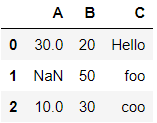Let’s access cell value of (2,1) i.e index 2 and Column B

``````df.at[2,'B']

30
``````

Value 30 is the output when you execute the above line of code

Now let’s update the only NaN value in this dataframe to 50 , which is located at cell 1,1 i,e Index 1 and Column A

``````df.at[1,'A']=50
df
``````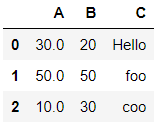So you have seen how we have updated the cell value without actually creating a new Dataframe here

Let’s see how do you access the cell value using loc and at

``````df.loc.B

OR

df.loc.at['B']

Output:
50
``````

Dataframe cell value by Integer position

From the above dataframe, Let’s access the cell value of 1,2 i.e Index 1 and Column 2 i.e Col C

iat - Access a single value for a row/column pair by integer position. Use iat if you only need to get or set a single value in a DataFrame or Series.

``````df.iat[1, 2]

Ouput
foo
``````

Let’s setup the cell value with the integer position, So we will update the same cell value with NaN i.e. cell(1,0)

``````df.iat[1, 0] = 100
``````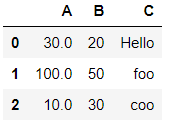Select rows in a MultiIndex Dataframe

Pandas xs Extract a particular cross section from a Series/DataFrame. This method takes a key argument to select data at a particular level of a MultiIndex.

Let’s create a multiindex dataframe first

``````#xs
import itertools
import pandas as pd
import numpy as np
a = ('A', 'B')
i = (0, 1, 2)
b = (True, False)
idx = pd.MultiIndex.from_tuples(list(itertools.product(a, i, b)),
names=('Alpha', 'Int', 'Bool'))
df = pd.DataFrame(np.random.randn(len(idx), 7), index=idx,
columns=('I', 'II', 'III', 'IV', 'V', 'VI', 'VII'))
``````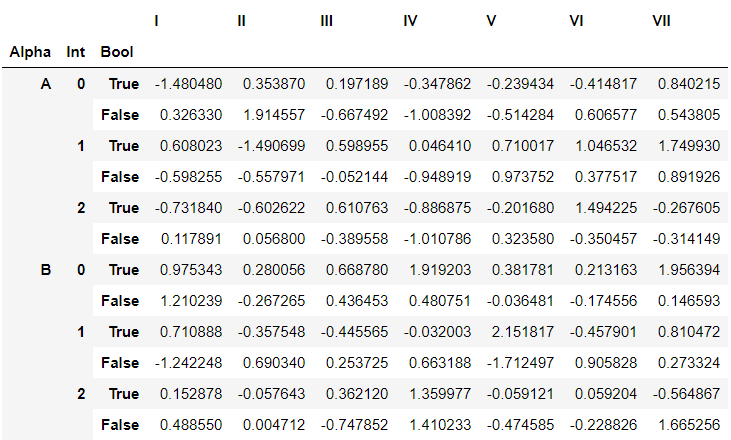Access Alpha = B

``````df.xs(('B',), level='Alpha')
``````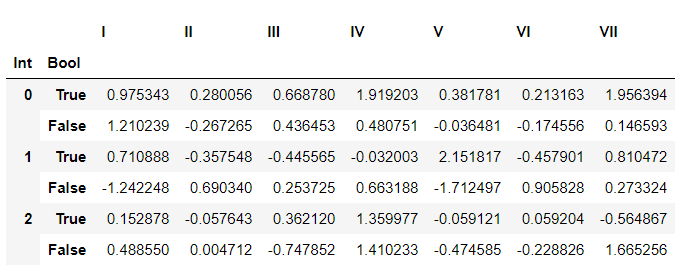Access Alpha = ‘B’ and Bool == False

``````df.xs(('B', False), level=('Alpha', 'Bool'))
``````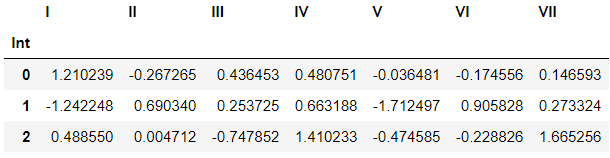Access Alpha = ‘B’ and Bool == False and Column III

``````df.xs(('B', False), level=('Alpha', 'Bool'))['III']
``````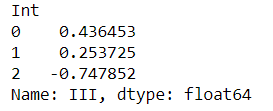Conclusion

So you have seen how you can access a cell value and update it using `at` and `iat which is` meant to access a scalar, that is, a single element in the dataframe, while `loc` and `iloc`are meant to access several elements at the same time, potentially to perform vectorized operations. at Works very similar to loc for scalar indexers. Cannot operate on array indexers.Advantage over loc is that this is faster. Similarly, iat Works similarly to iloc but both of them only selects a single scalar value. Further to this you can read this blog on how to update the row and column values based on conditions.

Tags:

Categories:

Updated: